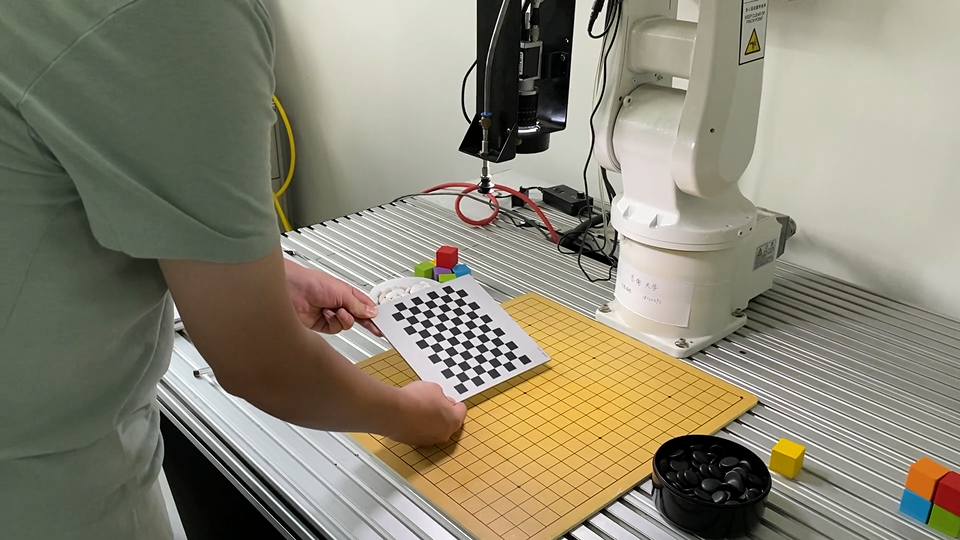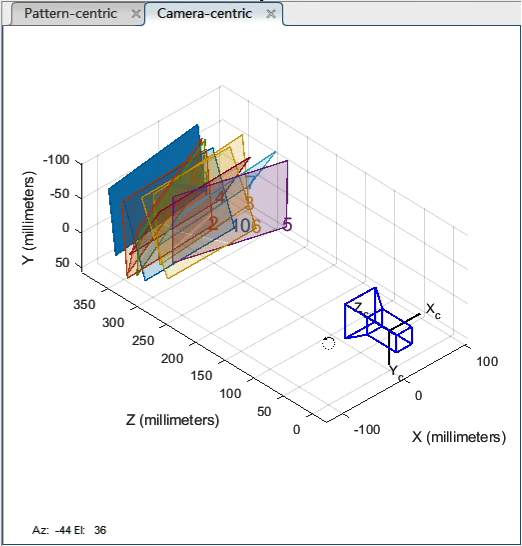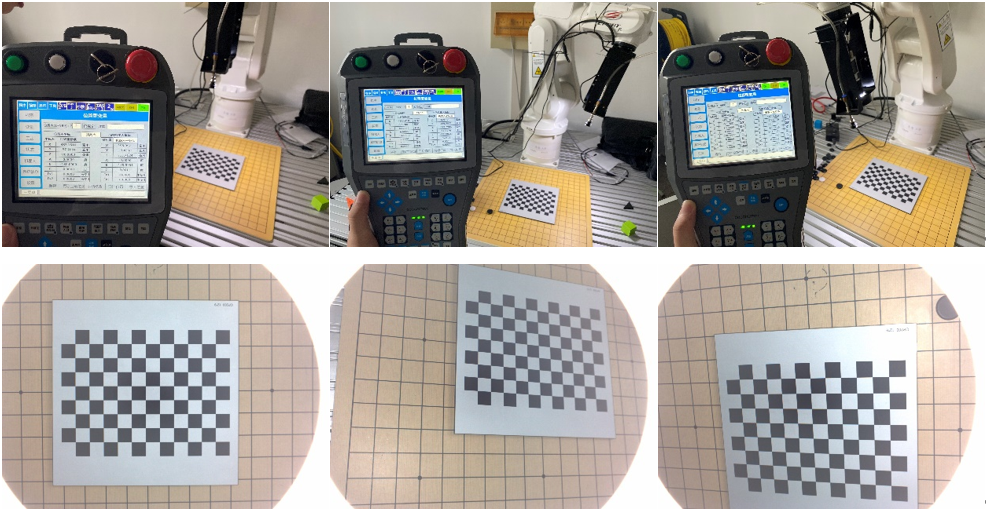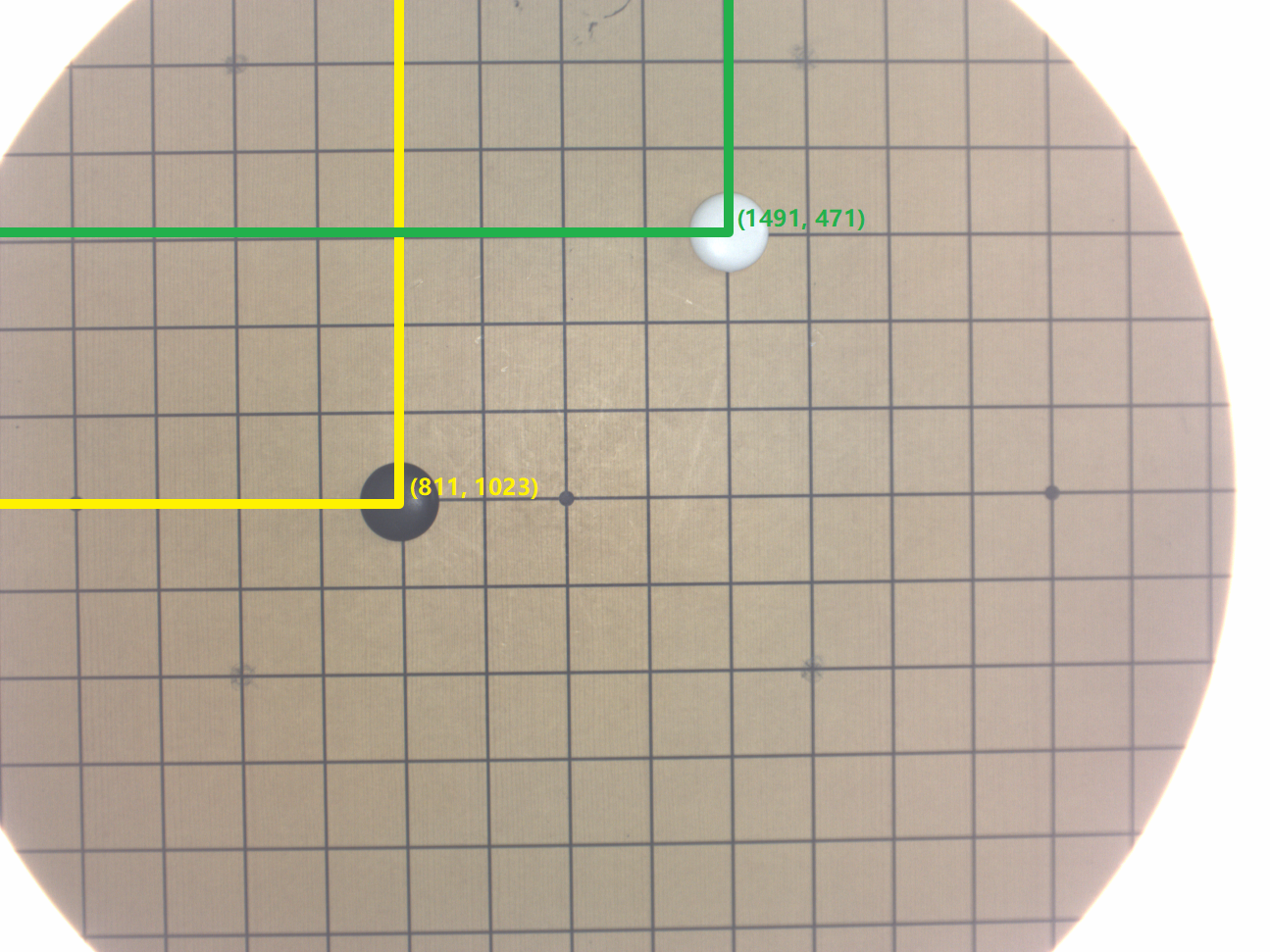## 硬件#

• 埃夫特 ER3A-C60
• 埃夫特紧凑型控制柜
• 固高示教器
• Basler acA1300-30gm 相机

## 前序知识#

（1）世界坐标系（Xw，Yw，Zw）：在环境中由用户任意选择一个基准坐标系作为参考来描述摄像机位置，在相机标定中一般选择标定板坐标系作为世界坐标系。 （2）摄像机坐标系（Xc，Yc，Zc）：以针孔模型的聚焦中心 O_c（光心）为原点，摄像机光轴为 Z 轴建立三维坐标系，其中 Xc轴、Yc轴与成像平面 x、y 轴平行。光心到图像平面的距离为有效焦距 f。 （3）成像平面坐标系（x，y）：光轴与成像平面交点，称为主点。成像平面坐标系以主点为原点，是以物理单位表示的平面直角坐标系。 （4）图像坐标系（u，v）：固定在图像上以像素为单位的平面直角坐标系，通常以图像左上角为像素原点，每一像素的（u，v）分别表示该像素在图像数组中的行数和列数。u、v 轴分别与 x、y 轴平行，该坐标系的单位为像素。

## 相机标定#改变标定板位姿可视化结果

## 手眼标定#

$$_b^wT=_g^wT _c^gT _b^cT$$

$_b^wT$为标定板相对于机器人的位姿关系，当标定板不动时，$_b^wT$为定量保持不变；$_g^wT$是执行器相对于机器人的位姿关系，由机器人控制器给出；$_c^gT$是摄像机相对于末端执行器的位姿关系，即为手眼标定所求量；$_b^cT$是标定板相对于摄像机的位姿关系，即摄像机标定所得的外参。

$${_g^wT_1} {_c^gT} {_b^cT_1}={_g^wT_2} {_c^gT} {_b^cT_2}$$手眼标定

$$\operatorname{Tch}=\left[\begin{array}{cccc}-0.0060 & -1.0427 & -0.0237 & 16.1604 \1.0158 & 0.0121 & 0.0144 & -44.7796 \0.0010 & -0.0240 & 1.0558 & 68.6513 \0 & 0 & 0 & 1.0000\end{array}\right]$$

$$H_1\cdot Tch\cdot C_1=\left[\begin{matrix}-0.0029&-1.0375&0.0124&455.0323\-1.0156&-0.0123&-0.0094&10.1779\0.0237&-0.0120&-1.0505&-31.8727\0&0&0&1.0000\end{matrix}\right]$$

$$H_2\cdot Tch\cdot C_2=\left[\begin{matrix}-0.1560&-0.9143&0.4673&373.8901\-0.8401&-0.1548&-0.5499&141.1848\0.5644&-0.4673&-0.7517&-82.9623\0&0&0&1.0000\end{matrix}\right]$$

## 定位#

$$\left[\begin{array}{l}X_{c} \Y_{c} \Z_{c}\end{array}\right]=\left[\begin{array}{ccc}f_{x} & 0 & u_{0} \0 & f_{y} & v_{0} \0 & 0 & 1\end{array}\right]^{-1} Z_{c}\left[\begin{array}{c}u \v \1\end{array}\right]$$

$$\begin{bmatrix}X_w \Y_w \Z_w \1\end{bmatrix}={_g^w}T\cdot {_c^g}T\cdot \begin{bmatrix}X_c \Y_c \Z_c \1\end{bmatrix}$$像素坐标系

$$\left[\begin{array}{c}398.2564 \-111.6166 \-9.9233\end{array}\right]$$